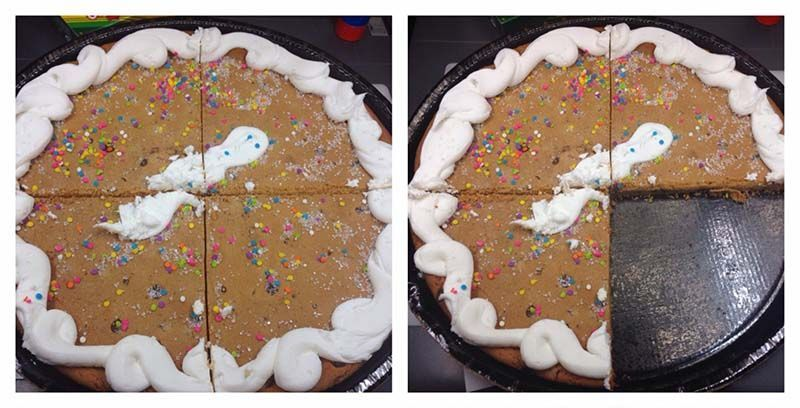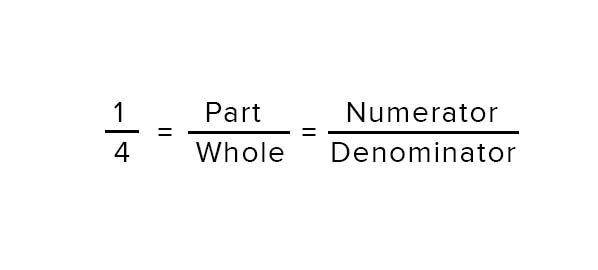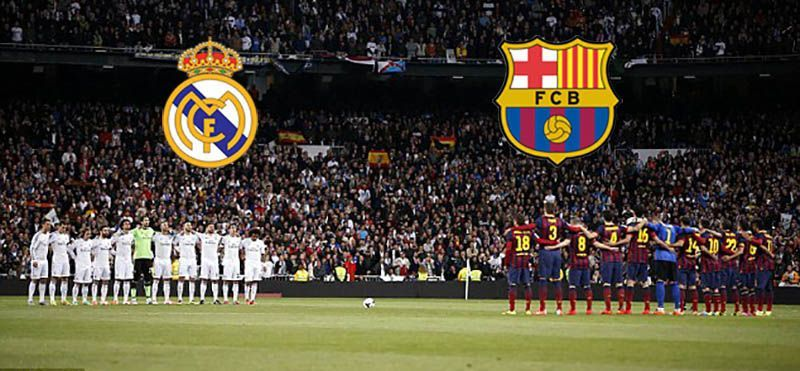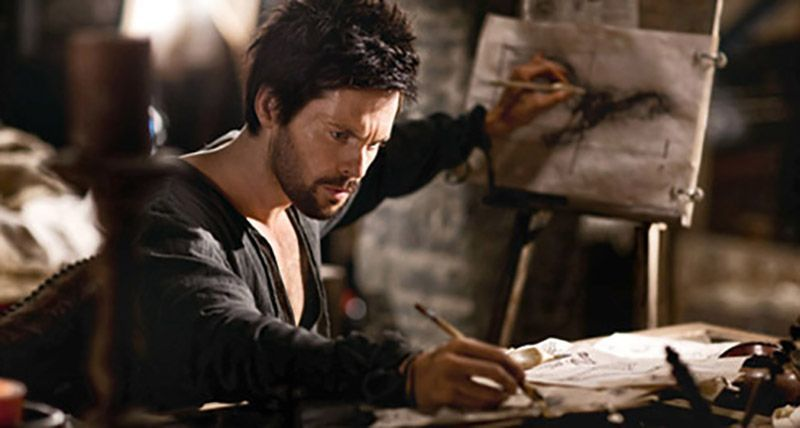# Frcations.A fraction simply tells us how many equal parts of a whole we have.

Let's watch a video to understand what fractions are.

# Fractions is. Making Parts.

Tom, Jerry and Spike are fighting because they didn't know how to divide the meat into equal parts.

Fractions help us divide things into equal parts, so that we don't fight like Tom, Jerry and Spike.

Fractions are formed when we divide a whole into equal parts.

Each equal part of the whole is called a fraction.To represent this part of the whole, we write it as 1 over 4.

Where the number above the horizontal line represents the parts and the number below the horizontal line represents the whole.

The number above the horizontal line which represents the parts is called the numerator.

And the number below the horizontal line which represents the whole is called the denominator.

# Access All Content

## More from Grade 4 Math# Pictograph

Representing your world in pictures! See how the type and number of a quantity can be mathematically represented pictorially.# Venn Diagram

The easiest way to imagine sets visually. See the power of Venn Diagrams.# Venn Diagram Example

Learn how to use Venn Diagrams to solve real world problems with these interesting examples.